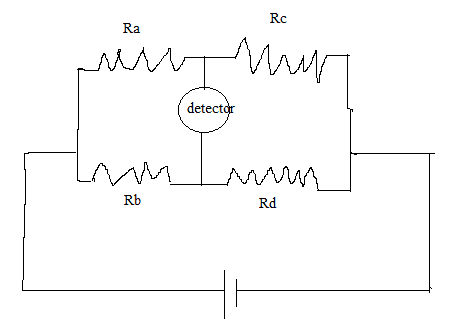# How to calculate detector voltage from resistances?

PhizKid

## Homework StatementThe resistances:
Ra = 10 ohm
Rb = 10 ohm
Rc = 10 ohm
Rd = 8 ohm

Battery = 10 volts

Voltage across detector = ?

## Homework Equations

V = IR
(series) R = R1 + R2 + ...
(parallel) 1 / R = (1/R1) + (1/R2) + ...

## The Attempt at a Solution

So to get the voltage, I need to find the total resistance and current. I tried to simplify the problem by calculating Ra and Rb in parallel, Rc and Rd in parallel, then adding up these resistances in series. So I get the total resistance to be:

Ra + Rb = ##\frac{1}{\frac{1}{10} + \frac{1}{10}}= 5## ohm
Rc + Rd = ##\frac{1}{\frac{1}{8} + \frac{1}{10}} = \frac{40}{9}## ohm

Ra + Rb + Rc + Rd = ##5 + \frac{40}{9} = \frac{85}{9}## ohm

So to get the current, I use V/R = I
Since battery is 10V:

##\frac{10}{\frac{85}{9}} = \frac{18}{17}A##

So now I need to find the voltage through this resistance, but V = RI doesn't work because I just get back the total voltage from the battery. Not sure what else to do here

## Answers and Replies

Mentor
Ra and Rb (and Rc and Rd) are not in parallel. The (ideal) voltage detector does not conduct current.

PhizKid
Is Ra and Rc (Rb and Rd) in series?

Mentor
Right.

PhizKid
OK, so if I add up the series first, can I then calculate the remaining 2 resistances in parallel?

Mentor
Sure.

willem2
OK, so if I add up the series first, can I then calculate the remaining 2 resistances in parallel?

You can. but it won't help. You have to calculate the voltage at the point between Ra and Rc, and at the point between Rb and Rd.

Ra and Rc form a voltage divider. You should know a formula for that . If yoiu don't, caculate the current throug Ra and Rc, and then the voltage drop across Rc.

Same thing for Rb and Rd

PhizKid
I'm not sure I understand the question. If there's no current through the detector, how can there be a voltage?